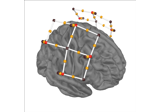# mne.preprocessing.nirs.scalp_coupling_index#

mne.preprocessing.nirs.scalp_coupling_index(raw, l_freq=0.7, h_freq=1.5, l_trans_bandwidth=0.3, h_trans_bandwidth=0.3, verbose=False)[source]#

Calculate scalp coupling index.

This function calculates the scalp coupling index . This is a measure of the quality of the connection between the optode and the scalp.

Parameters:
rawinstance of `Raw`

The raw data.

l_freq

For FIR filters, the lower pass-band edge; for IIR filters, the lower cutoff frequency. If None the data are only low-passed.

h_freq

For FIR filters, the upper pass-band edge; for IIR filters, the upper cutoff frequency. If None the data are only high-passed.

l_trans_bandwidth

Width of the transition band at the low cut-off frequency in Hz (high pass or cutoff 1 in bandpass). Can be “auto” (default) to use a multiple of `l_freq`:

```min(max(l_freq * 0.25, 2), l_freq)
```

Only used for `method='fir'`.

h_trans_bandwidth

Width of the transition band at the high cut-off frequency in Hz (low pass or cutoff 2 in bandpass). Can be “auto” (default in 0.14) to use a multiple of `h_freq`:

```min(max(h_freq * 0.25, 2.), info['sfreq'] / 2. - h_freq)
```

Only used for `method='fir'`.

verbose

Control verbosity of the logging output. If `None`, use the default verbosity level. See the logging documentation and `mne.verbose()` for details. Should only be passed as a keyword argument.

Returns:
sci

Array containing scalp coupling index for each channel.

References

## Examples using `mne.preprocessing.nirs.scalp_coupling_index`#Preprocessing functional near-infrared spectroscopy (fNIRS) data

Preprocessing functional near-infrared spectroscopy (fNIRS) data